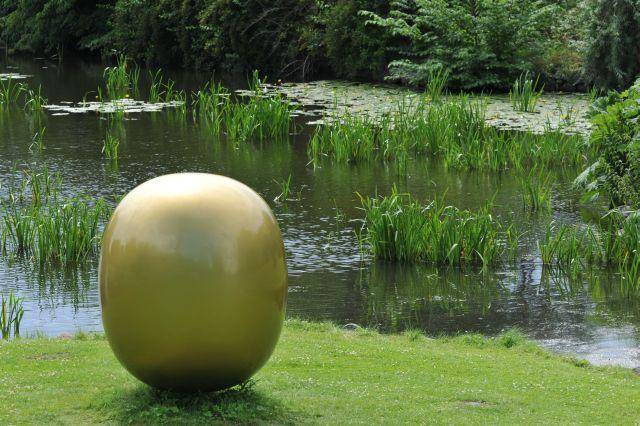# Hein's Egg

Calculus Level 5The poet and scientist Piet Hein created an egg that will stand on its oblong edge. He called it the superegg, which is a solid of revolution (about the y-axis) characterized by the equation ${ \left( \frac { x }{ a } \right) }^{ 2.5 }+{ \left( \frac { y }{ b } \right) }^{ 2.5 } =1.$

Calculate the volume of the superegg if $a = 2$ and $b = 3$.

×

Problem Loading...

Note Loading...

Set Loading...